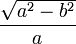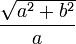# Eccentricity

Eccentricity: how much a conic section (a circle, ellipse, parabola or hyperbola)
varies from being circular.

A circle has an eccentricity of zero, so the eccentricity shows you how "un-circular" the curve is. Bigger eccentricities are less curved.Different values of eccentricity make different curves:

• At eccentricity = 0 we get a circle
• for 0 < eccentricity < 1 we get an ellipse
• for eccentricity = 1 we get a parabola
• for eccentricity > 1 we get a hyperbola
• for infinite eccentricity we get a line

Eccentricity is often shown as the letter e (don't confuse this with Euler's number "e", they are totally different)

## Animation

Try the slider to see what happens:

## Calculating The ValueFor a circle, eccentricity is 0For an ellipse, eccentricity is:For a parabola, eccentricity is 1For a hyperbola, eccentricity is: